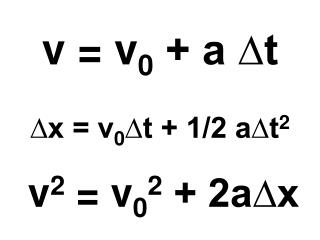DownloadDownload Presentationv = v 0 + a ∆t ∆x = v 0 ∆t + 1/2 a∆t 2 v 2 = v 0 2 + 2a∆x

# v = v 0 + a ∆t ∆x = v 0 ∆t + 1/2 a∆t 2 v 2 = v 0 2 + 2a∆x

Download Presentation## v = v 0 + a ∆t ∆x = v 0 ∆t + 1/2 a∆t 2 v 2 = v 0 2 + 2a∆x

- - - - - - - - - - - - - - - - - - - - - - - - - - - E N D - - - - - - - - - - - - - - - - - - - - - - - - - - -
##### Presentation Transcript

1. v = v0 + a ∆t∆x = v0∆t + 1/2 a∆t2v2 = v02 + 2a∆x

2. To create equations for freely falling bodies, simply replace a with g.

3. v = v0 + a ∆t∆x = v0∆t + 1/2 a∆t2v2 = v02 + 2a∆x

4. v = v0 + g ∆t∆x = v0∆t + 1/2 g∆t2v2 = v02 + 2g∆x

5. When freely falling bodies start from rest, v0 = 0, so:v = g ∆t∆x = 1/2 g∆t2v2 = 2g∆x

6. These equations refer to a situation with constant acceleration due to gravity and no air resistance. Such motion is called free fall.

7. Example: A ball is dropped froma height of 10 meters. How long will it be in the air before it strikes the floor?

8. Example: A ball is thrown vertically upward with a velocity of 100 m/s. (a) To what height will it rise? (b) How long will it take for the ball to fall back to the earth?

9. Example: A ball drops from rest and attains a velocity of 62 m/s. How much time has elapsed?

10. Example: How far did the ball in the previous problem fall during the third second?